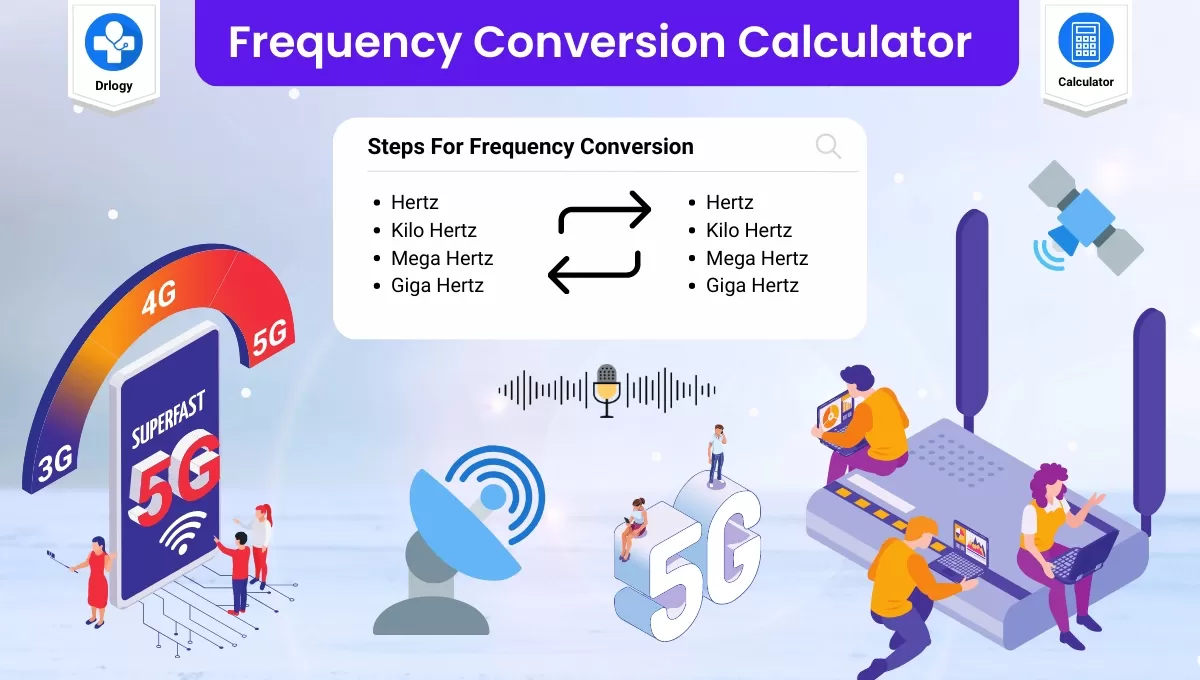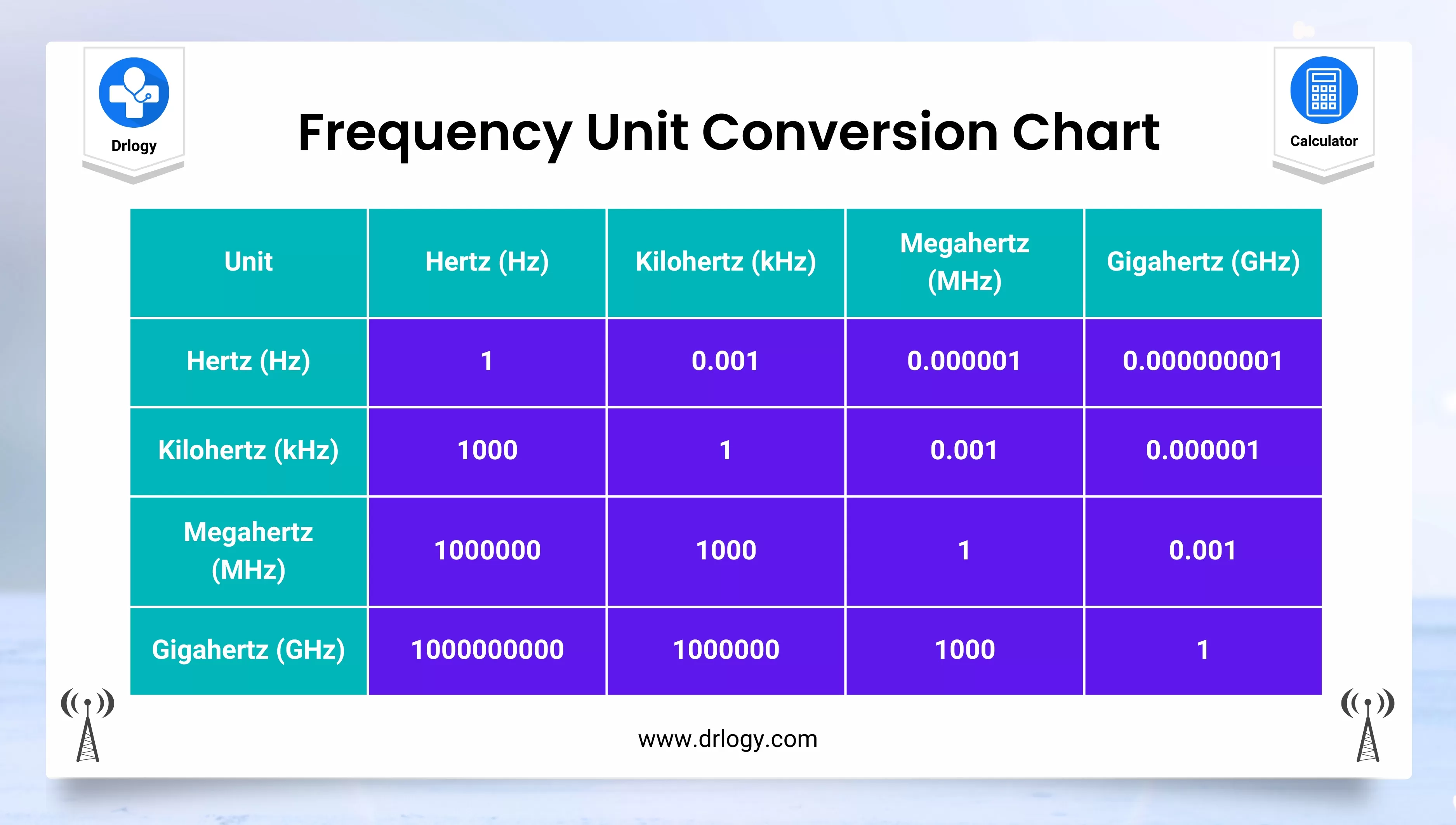• Health
• Pet
• Unit Conversion
• Medical# Frequency Conversion Calculator Formula And Chart

## Frequency Conversion Calculator

Result : =
keyboard_arrow_down
=
keyboard_arrow_down

## Frequency Conversion Calculator Overview

• A frequency conversion calculator is a tool used to convert a frequency measurement from one unit to another.
• The most common frequency units include hertz (Hz), kilohertz (kHz), megahertz (MHz), and gigahertz (GHz).
• Frequency conversion refers to the process of changing the frequency of a signal from one frequency to another frequency. This process is commonly used in various fields, such as electronics, telecommunications, and broadcasting.
• Frequency conversion is achieved through the use of frequency conversion devices, such as mixers, amplifiers, and filters. These devices can modify the frequency of a signal through processes such as frequency multiplication, frequency division, and frequency mixing.
• To use a frequency conversion calculator, simply enter the value you wish to convert and select the units you want to convert from and to. The calculator will then provide you with the converted value.
• It is important to note that frequency conversions are based on a multiplication factor, where higher frequency units are a multiple of lower frequency units. For example, 1 MHz is equal to 1,000 kHz, which is equal to 1,000,000 Hz.

## Steps For Frequency Conversion Calculator

Here are the steps to use a frequency conversion calculator:

1. Determine the frequency value you want to convert.
2. Determine the unit of measurement for the frequency value you want to convert.
3. Determine the unit of measurement you want to convert the frequency value to.
4. Find a frequency conversion calculator online or use a physical calculator that has the conversion functions.
5. Input the frequency value you want to convert into the calculator.
6. Select the unit of measurement for the frequency value you want to convert from.
7. Select the unit of measurement you want to convert the frequency value to.
8. The calculator will display the converted frequency value in the selected unit of measurement.
9. Double-check the converted frequency value to ensure accuracy.

It's important to note that frequency conversion calculators are based on multiplication factors. Therefore, it's important to know the conversion factor between the units of measurement. Some common conversion factors have been provided in the other answers to this question.

## Frequency Conversion Calculator Units

Frequency conversion calculators can convert frequencies between various units of measurement. The most common units used in frequency conversion calculators include:

1. Hertz (Hz): The base unit of frequency measurement, representing one cycle per second.
2. Kilohertz (kHz): One kilohertz is equal to 1,000 hertz.
3. Megahertz (MHz): One megahertz is equal to 1,000,000 hertz.
4. Gigahertz (GHz): One gigahertz is equal to 1,000,000,000 hertz.

Other less common units of frequency measurement include terahertz (THz), millihertz (mHz), and microhertz (µHz). Some frequency conversion calculators may also support the conversion of frequencies in these units. However, for most practical purposes, the four units mentioned above are sufficient.

## Frequency Conversion Calculator Formula

The formula to convert a frequency value from one unit to another depends on the specific conversion you are trying to perform. However, the general formula to convert a frequency value from one unit to another is:

Converted Frequency = (Input Frequency x Conversion Factor)

where:

• Input Frequency is the original frequency value you want to convert.
• Conversion Factor is the factor used to convert between the two units of measurement.

For example, to convert a frequency of 50 MHz to kHz, you would use the conversion factor of 1000, since there are 1000 kHz in 1 MHz. Therefore, the formula would be:

50 MHz x 1000 = 50,000 kHz

So, 50 MHz is equal to 50,000 kHz.

It's important to note that some conversion factors are not multiples of 10, so the formula may differ depending on the units being converted. Additionally, some calculators may have additional steps or formulas for more complex frequency conversions.

## Frequency Conversion Calculator Chart

Here is a frequency conversion chart that shows the most common frequency units and their conversion factors:

Unit Symbol Conversion Factor
Hertz Hz 1
Kilohertz kHz 1,000
Megahertz MHz 1,000,000
Gigahertz GHz 1,000,000,000
• To convert from one unit to another, simply multiply or divide by the conversion factor.
• For example, to convert 2 GHz to Hz, you would multiply 2 by 1,000,000,000, resulting in 2,000,000,000 Hz.
• To convert 500 kHz to MHz, you would divide 500 by 1,000, resulting in 0.5 MHz.
• It is important to note that some calculators may use slightly different conversion factors, so always double-check your results to ensure accuracy.## Frequency Conversion Calculator Table

Here is a table that shows the conversion factors for frequency units:

Convert from ↓\ To → Hertz Kilohertz Megahertz Gigahertz
Hertz (Hz) 1 0.001 0.000001 0.000000001
Kilohertz (kHz) 1000 1 0.001 0.000001
Megahertz (MHz) 1000000 1000 1 0.001
Gigahertz (GHz) 1000000000 1000000 1000 1

• To use the table, locate the row of the unit you want to convert from and the column of the unit you want to convert to. The intersection of the row and column will give you the conversion factor.
• For example, to convert 2 GHz to MHz, locate the row for GHz and the column for MHz. The intersection is 1000, so you would multiply 2 by 1000 to get 2000 MHz.

## Frequency Conversion Calculator Benefits

Frequency conversion can be used for various purposes, such as to change the frequency of a signal for transmission, to improve signal quality, to reduce interference, or to generate new signals at different frequencies.

Here are some benefits of using a frequency conversion calculator:

1. Accuracy: Frequency conversion calculators provide accurate conversion results, which can be helpful in situations where precise frequency measurements are necessary.
2. Time-saving: Using a frequency conversion calculator can save time by quickly converting frequencies from one unit to another, without the need for manual calculations.
3. Easy to use: Most frequency conversion calculators are user-friendly and easy to use, requiring only a few steps to convert frequencies.
4. Versatility: Frequency conversion calculators can convert frequencies between different units, making them versatile tools for use in a variety of fields such as electronics, telecommunications, and engineering.
5. Eliminates errors: Manual calculations can sometimes lead to errors, especially when dealing with complex frequency conversions. Using a frequency conversion calculator helps to eliminate such errors and provide accurate results.
6. Portable: Frequency conversion calculators are often portable and can be easily carried around, making them convenient tools for use in the field or on the go.

Summary

Overall, using a frequency conversion calculator is a quick and easy way to convert frequencies between different units, ensuring accuracy and saving time. Check more unit conversion calculators like this to solve your daily problems on Drlogy Calculator to get exact solution.

Reference

• Frequency conversion By Wikipedia .
• Frequency conversions Tables .
favorite_border 3526 Likes

## Frequency Conversion Calculator FAQ

### What is the formula of frequency of conversion?

The formula for frequency conversion depends on the specific type of conversion you want to perform. However, the general formula for frequency conversion is:

f2 = f1 x (N2 / N1)

where:

• f1 is the frequency of the input signal.
• f2 is the frequency of the output signal.
• N1 is the number of cycles in the input signal.
• N2 is the number of cycles in the output signal.

This formula is commonly used for frequency conversion when the number of cycles in the input and output signals are different.

For example, if you want to convert an input signal with a frequency of 10 MHz to an output signal with a frequency of 20 MHz, the formula would be:

f2 = f1 x (N2 / N1) f2 = 10 MHz x (2 / 1) f2 = 20 MHz

So, the frequency of the output signal would be 20 MHz.

• It's important to note that this formula is just one example of a frequency conversion formula, and other formulas may be used depending on the specific type of conversion being performed.

### How do you convert frequency units?

To convert frequency units, you can use a frequency conversion calculator or follow these steps:

1. Determine the frequency value you want to convert.

2. Determine the unit of measurement for the frequency value you want to convert from.

3. Determine the unit of measurement you want to convert the frequency value to.

4. Use the appropriate conversion factor to convert between the two units of measurement.

5. Multiply the frequency value by the conversion factor.

6. The result will be the frequency value in the desired unit of measurement.

### How do I calculate my frequency?

The frequency of a signal can be calculated using the formula:

f = 1 / T

where:

• f is the frequency of the signal in hertz (Hz).
• T is the period of the signal in seconds (s).

The period is the amount of time it takes for one cycle of the signal to occur. To calculate the period, you can use the formula:

T = 1 / f

where:

• T is the period of the signal in seconds (s).
• f is the frequency of the signal in hertz (Hz).

So, if you have a signal with a period of 0.02 seconds, the frequency would be:

f = 1 / T f = 1 / 0.02 f = 50 Hz

Therefore, the frequency of the signal would be 50 Hz.

View More Health

## Medical Calculator

View More Medical

View More Pet

## Unit Conversion Calculator

View More Unit Conversion

## Financial Calculator

View More Financial
• Home >
• Calculator >
• Frequency Conversion Calculator Formula And Chart
Drlogy®
The Power To Health